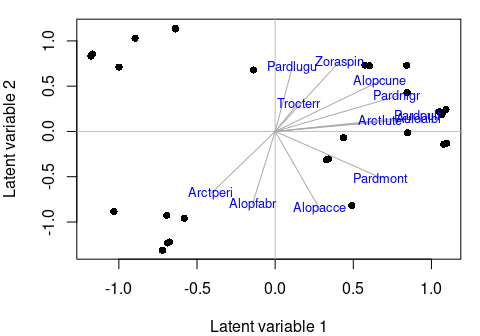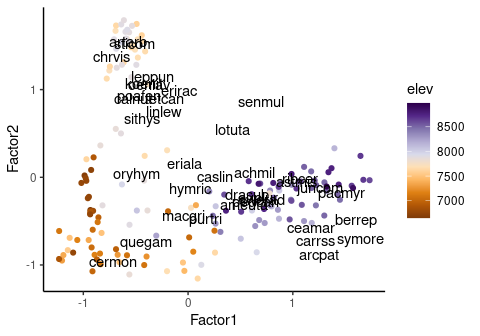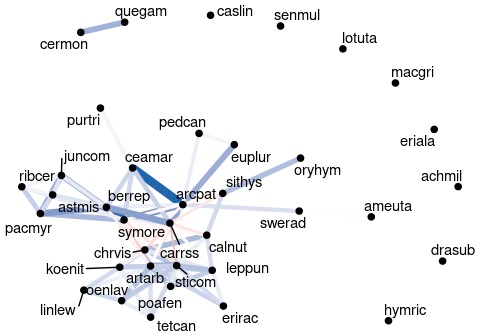# ecoCopula package for model based ordination and graphical modelling in ecology

## Overview

The ecoCopula package allows you to visualise multivariate discrete data with graphical models and ordination. The package was designed primarily for multivariate abundance data in ecology, however it can be applied to any multivariate discrete data.

The two main functions are:

1. Copula ordination (cord) to visualise how samples (sites) and variables (taxa) are located along several latent variables (unobserved environmental gradient).
2. Copula graphical models (cgr) to plot a graph which distinguishes between direct and indirect associations between variables (e.g taxa).

The ecoCopula package used model-based methods (rather than distance-based, e.g. nMDS). Both cord and cgr will work on either a stackedsdm object (stacked species distribution model, from ecoCopula) or a manyglm object (from mvabund).

## Under the hood

### Copulas for discrete data

Copulas are a way to construct a multivariate distribution, and can be used as an alternative to hierarchical models (as in gllvm, HMSC, boral) and generalised estimating equations (GEE; as in mvabund). Like hierarchical models, but unlike GEEs, copulas directly model covariance between variables (taxa). The main advantage of copulas relative to hierarchical models is speed, with copula ordination usually being at least 10 times faster than ordination with hierarchical models, and for large sample sizes faster non-metric multidimensional scaling (nMDS). For more details about copulas and how models are fit in ecoCopula see Popovic et. al. (2018,2019).

### Copula ordination

Model based ordination methods, including copula ordination using the cord function as well as gllvm, boral and HMSC, are implemented with latent variables. These can be interpreted as unobserved environmental covariates. Biplots are created by plotting the scores of sites and loadings of taxa. By directly modelling the data, model-based methods account for both the natural variation mean-variance relationships in multivariate abundance data. We can use standard statistical tools to check the assumptions and perform model selection, quantify the uncertainty in the estimated correlations between taxa, and predict to existing and/or new sites, all of which are generally more challenging when using dissimilarity-based ordination methods (like nMDS).

### Copula graphical models

Graphical models look at direct and indirect associations between variables (taxa). They try to answer the question: Is the abundance of this pair of taxa related, after accounting for the effect of the abundances of all the other taxa in the data? A simple and crude way to do this might be regressing each of the two taxa separately (as response) against all the other taxa in the data (as predictors), and then looking for any residual correlation between the pair. Graphical models do something like this, but using modern statistical techniques to improve efficiency and model the community jointly.

Install ecoCopula in the usual way.

install.packages("ecoCopula")

Then attach the library.

library(ecoCopula)
#> Loading required package: mvabund

## Hunting spiders (counts and presence/absence)

The hunting spider dataset (van der Aart & Smeenk-Enserink, 1975) has counts of 12 hunting spiders at 28 sites, collected using pit traps, as well as 6 environmental covariates. Species codes are first four letters of genus then first four letters of species. We will analyse it both as counts and presence-absence.

# spider data is stored in ecoCopula
data(spider)
X <- as.data.frame(spider$x) # environmental covariates Counts abund <- spider$abund # abundance of spiders
abund[1:5,1:6]
#>      Alopacce Alopcune Alopfabr Arctlute Arctperi Auloalbi
#> [1,]       25       10        0        0        0        4
#> [2,]        0        2        0        0        0       30
#> [3,]       15       20        2        2        0        9
#> [4,]        2        6        0        1        0       24
#> [5,]        1       20        0        2        0        9

Presence-absence

pa=(abund>0)*1 # presence-absence of spiders
pa[1:4,1:6]
#>      Alopacce Alopcune Alopfabr Arctlute Arctperi Auloalbi
#> [1,]        1        1        0        0        0        1
#> [2,]        0        1        0        0        0        1
#> [3,]        1        1        1        1        0        1
#> [4,]        1        1        0        1        0        1

### Ordination

To plot an ordination biplot for presence-absence data pa, we first fit a marginal model using stackedsdm or manyglm with family="binomial", then use the copula ordination function cord on the resulting object, and plot the output.

# fit marginal model
spider_pa <- stackedsdm(pa,~1, data = X, family="binomial",ncores = 2) #eqiv. manyglm()
# fit copula ordination
spid_lv=cord(spider_pa)
# biplot
plot(spid_lv,biplot = TRUE)To colour the sites by a predictor, we can create a colour variable.

sp_res <- data.frame(bryce_ordi$loadings, species = colnames(bryceord)) alpha= 2.5 # scaling parameter ggplot()+ geom_point(aes(x=Factor1,y=Factor2,color = elev ),site_res)+ #sites geom_text(aes(x = Factor1*alpha, y = Factor2*alpha,label = species),data=sp_res)+ #species scale_color_gradientn(colours = brewer.pal(n = 10, name = "PuOr"))+ theme_classic()Low elevation and high elevation sites have quite different species, with low elevations associated with e.g. cermon, and high elevation with e.g. pacmyr. ### Graphical model of veg species We will control for slope. cont_preds=sapply(brycesite, class)%in%c("int","num") brycesite[,cont_preds]=scale(brycesite[,cont_preds]) bryce_marg_all <- stackedsdm(bryceord, formula_X = ~ slope, data = brycesite, family="ordinal",ncores = 2) bryce_graph<-cgr(bryce_marg_all, seed = 1) #seed for demonstration For more detailed plots cgr has an igraph output, which you can manipulate and plot with the tidygraph and ggraph packages as well as the igraph package. igraph_out<-bryce_graph$best_graph$igraph_out library(tidyr) library(tidygraph) library(ggraph) igraph_out %>% ggraph('fr') + # see ?layout_tbl_graph_igraph geom_edge_fan0(aes( colour = partcor, width=partcor)) + scale_edge_width(range = c(0.5, 3))+ scale_edge_color_gradient2(low="#b2182b",mid="white",high="#2166ac")+ geom_node_text(aes(label=name), repel = TRUE)+ geom_node_point(size=2)+ theme_void() + theme(legend.position = 'none')There is a strong positive association between e.g. ceamar and arcpat after controlling for all the other species. ## Other ecoCopula functionality and notes • stackedsdm can take a vector of families, this means each column can have a different distribution. This is most often useful when modelling traits rather than abundance (some of which are binary, and some continuous), but could also be useful if you have abundance data collected differently for different taxa (some presence/absence, some counts, some ordinal) • Residual ordination: by including predictors in the stackedsdm or manyglm model, you are controlling for these. Any ordination you will control for the effect of these variables. This might make it easier to see the effect of other variables. • It is not uncommon for cgr to output a graph with no edges. The final model you plot is the best model chosen by BIC. With a small amount of data this is often a model with no associations. The models are indexed by the shrinkage parameter lambda, and given graphical modelling is exploratory, you can further explore different models by changing lambda manually. You can find the shrinkage parameter for the best model with cgr_fit$all_graphs\$lambda.opt where cgr_fit is your fitted cgr object. Smaller values of lambda give more dense graphs with more edges. You can then fit a model with a smaller lambda with the lambda argument in cgr.

• Both cord and cgr have a n.samp argument. If you find different runs of these functions give different results, try increasing n.samp.